Abstract. Mathematician Jay Kappraff discusses a pair of tables of integers found in the Nicomachus's Introduction to Arithmetic and shows how they lead to a general theory of proportion. He shows how the system of musical proportions developed by the neo-Platonic Renaissance architects Leon Battista Alberti and Andrea Palladio, the Roman system of proportions described by Theon of Smyrna, and the Modulor of Le Corbusier are derived naturally from the Nicomachus tables.

#The Arithmetic of Nicomachus of Gerasa
and its Applications to Systems of Proportion

Jay Kappraff
New Jersey Institute of Technology
Department of Mathematics
University Heights
Newark, New Jersey 07102 USA

INTRODUCTION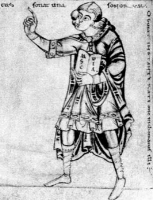N
icomachus of Gerasa (Figure 1, at left) has gained a position of importance in the history of ancient mathematics due in great measure to his Introduction to Arithmetic . This book is one of the only surviving documentations of Greek number theory. Little is known about the life of Nicomachus, and the period of his life can only be estimated to lie between the middle of the first century and the middle of the second century AD, making him contemporary with Theon of Smyrna and Ptolemy. I will discuss a pair of tables of integers found in the Arithmetic and show how they lead to a general theory of proportion. These include the system of musical proportions developed by the neo-Platonic Renaissance architects Leon Battista Alberti and Andrea Palladio, the Roman system of proportions described by Theon of Smyrna, and the Modulor of Le Corbusier. My interest in this article is to show how all of these systems are derived naturally from the Nicomachus tables. Although the architectural details have been discussed elsewhere, I will describe the Roman system of proportions in some depth.

This essay should not be looked at as an historical study. M.L. D'Ooge  has admirably presented Nicomachus's treatise in an historical context, and I have nothing to add. Rather I shall take a single theme found in the Arithmetic and look at it with the lens of a mathematician. Nicomachus also wrote another surviving manuscript, The Manual of Harmonics , and one of his tables leads naturally to a representation of the musical scale of Pythagoras. However, I will leave the discussion of such matters to a future article.

NICOMACHUS SEQUENCES
The following pair of double geometric series appear in the Introduction to Arithmetic and will be referred to as Nicomachus sequences: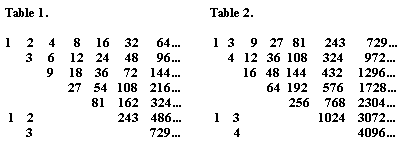These tables follow a kind of Pascal's law in which each number is the sum of the two above it. Note that in Table 1 each column ends when the integer is no longer divisible by 2, while the second table ends when integers are no longer divisible by 3. The motif of each table is expressed by the triangle of numbers shown to the left. Successive elements in the rows of Table 1 (Table 2) are in the ratio of 1:2 (1:3) while rows successive elements in each column are in the ratio of 2:3 (3:4). Also successive numbers on right leaning diagonals are in the ratio 4:3 (9:4).

Tables 1 and 2 are generalized to any real number x as shown in Table 3.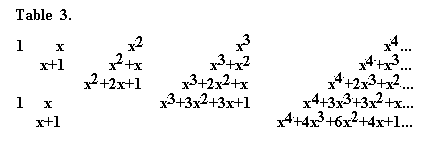Again each column ends when an element is not divisible by x. The terms of the geometric series on the diagonal are seen to be (1+x)n for n=0,1,2,… The triangle of numbers, 1, x, x+1 can be looked at as the transformation,

x:1 ® 1:(1+x) and x:(1+x)

Nicomachus actually thought of this relationship as a transformation and says in Chapter XXIII of Book I,

The multiple superpartients* and superpartients of other kinds are made to appear --
out of the superpartients; for example, from the superbipartient relation arranged so
as to begin with the smallest term (5:3 or 1 + 2:3) comes the double superbipartient
(8:3 or 2 + 2:3), but arranged so as to start with the greatest, the superpartient ratio
(8:5 or 1 + 3:5). Thus from (ratio x:1 = 3:5) comes either 25,40,64 (ratio 1:(1+x) = 5:8)
9,15,25 or 9,24,64 (ratio x: (1+x) = 3:8).

(*In order to represent fractions, Greek arithmetic employed a system in which ratios were referred to as : multiples, mn:n; superparticulars, as 1 + 1:n; superpartients, as 1 + k:n, k>1; multiple superparticulars, as m + 1:n, m>1; multiple superpartients, as m + k:n, both m and k being greater than 1.)

He then goes on to cite another example: From 16, 28, 49 (ratio 4:7) comes either 16, 44, 121 (ratio 4:11) or 49,77,121 (ratio 7:11).

Apart from the archaic language, this transformation appears to relate to the Farey sequence shown in Table 4. Farey sequences are, in turn, related to representations of rational numbers as continued fractions with applications to dynamical systems [3,4]. They also have applications to musical tuning systems [5,6]. The numerator (N) and denominator (D) of any rational number in the Farey sequence is gotten by adding the numerators and denominators of the two terms that brace it from the rows above it, e.g., 3/5 is braced by ½ and 2/3 so 3/5 = (1+2)/(2+3). In the Farey sequence each rational number x = N/D in one row gives rise to two rationals, 1: (1+x) = D/(N+D) and x:(1+x) = N/(N+D) in the next row, e.g., 2:5 ® 2:7 and 5:7. In this sense, Nicomachus indicates an early awareness of the concepts underlying the Farey sequence which has become an important tool of modern mathematics and music.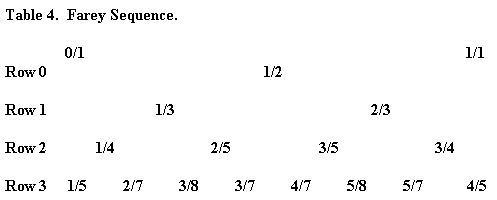...

Figure 2 indicates a geometrical construction leading from x to 1 : (1+x) and x : (1+x). A line is drawn from the lower left hand corner of a rectangle to the point x = N/D on a number line superimposed on the top edge of the rectangle. At the point where this line crosses the diagonal draw a vertical line. The vertical line marks the number line at x/(1+x) = N/(N+D). Next rotate Figure 1 by a halfturn; the vertical line intercepts a number line on the rotated edge of the rectangle at 1/(1+x) = D/(N+D). This construction is also inherent in the ability of the eight-pointed star discovered by Tons Brunés [7,8] and illustrated in Figure 3, to divide lines into 2,3,4,5,…,8 equal line segments. Brunés claims that he saw this star in a temple in Pompeii that was dated sometime in the first century AD. Is it possible that Nicomachus was aware of this star?

A SYSTEM OF PROPORTION BASED ON NICOMACHUS'S SEQUENCES
If Tables 1 and 2 are rewritten with elements in rows past the first shifted one element to the right, notice that each element of a given row has a value between the values of the two elements bracing it from above.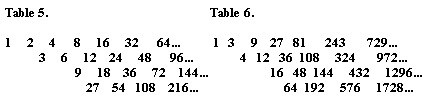Table 5 nicely reflects the three means that pervade mathematics, the geometric, arithmetic, and harmonic means. Given the sequence: a c b,

the geometic mean of a and b is c = Öab
the arithmetic mean is defined as: b-c = c-a or c = (a+b)/2
the harmonic mean is defined as : (b-c)/b = (c-a)/a or c = 2ab/(a+b).

Notice in Table 5 that each element is the geometric mean of the elements to the left and right of it; an element of a given row is the arithmetic mean of the two elements that brace it from above; while a given element fits between the two numbers in the row below as the harmonic mean.

What about the mean relations expressed by Table 6? Again each row is a geometric progression and again each element of a given row fits between the two numbers in the row above and below. However, an element of a given row is now a kind of generalized arithmetic mean defined by c=(a+b)/3 while a given element fits between two numbers in the row below it as a generalized harmonic mean c = 3ab/(a+b). These mean relations can be generalized to Table 3 where now the generalized arithmetic mean ca is defined as ca = (a+b)/x and the harmonic mean ch as ch=xab/(a+b). However, there is now an important restriction. In order for the means to lie between their respective pairs of numbers, x must satisfy the inequality x>f where f = (1+Ö5)/2, the golden mean. This result can be stated as a theorem (the proof is given in the Appendix). I will refer to this as Nicomachus's Theorem although Nicomachus was certainly not aware of the golden mean as we think of it today.

NICOMACHUS'S THEOREM:

If b = ax and x ³ f, then a £ ca < b and a < ch £ b,

where ca = (a + b)/x and ch = xab/(a + b).

For the elements in Table 2, we can define another table in which each element is the common (not generalized) arithmetic mean of the two elements above it while each element is the common harmonic mean of the two elements below it as shown in Table 7.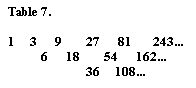A similar table for the geometric series based on 1:x can be created, although the table for x=2 is, of course, identical with the original and the table for x = f collapses into a single row with the other rows repetitions of this row.

There is one noteworthy relation tying the common and generalized means for a given x together. Place the corresponding arithmetic and harmonic means, both common and generalized, between any pair of elements from Table 1 and 2, e.g., the sequence of common and generalized means between 6 and 12 for Table 1 and 12 and 36 for Table 2 are: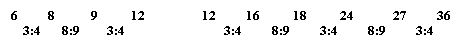The ratio of successive integers in this sequence are written below, and the product of these ratios equals 1/2 for the 1:2 sequence and 1/3 for the 1:3 sequence. In a similar manner, it can be shown that the product of the ratios for a 1:x sequence is 1/x.

ALBERTI'S SYSTEM OF MUSICAL PROPORTIONS
Any system of proportions useful for architecture should have additive properties so that the parts of a plan can be made to add up to the whole, and it should have a repetition of key ratios so that the design exhibits harmony in which the various parts relate to each other. We have seen that Nicomachus's tables all have additive properties. And while it might appear speculative that Alberti based his proportions on this classic mathematician, we know for a fact that Alberti held Nicomachus in great esteem, holding him up as an example of a great mathematician in his treatise De re aedificatoria [18, p.317].

Table 5 was the basis of the system of musical proportions used by Leon Battista Alberti in Renaissance Italy ,. The ratio of numbers in the rows of Table 5 are in proportion 1:2 which represents the musical octave. Depressing the string of a monochord at its midpoint and sounding it with a bow yields a tone one octave above the fundamental tone characteristic of the whole string (see Figure 4). The integers on the left leaning diagonal are in the ratio 2:3 representing the musical fifth, i.e., if the fundamental is a C, the fifth is a G (five tones from C to G [CDEFG]), while the integers on the right leaning diagonal are in the ratio 3:4, a musical fourth, i.e., if the fundamental is C the fourth is an F (CDEF). The fifth and fourth correspond to bowed string lengths of 2/3 and 3/4 of the length of the fundamental as shown in Figure 4. Alberti's system of proportions was built upon the neo-Platonic ideas of the times in which the pair of geometric series framing the edges of Table 5 correspond to Plato's lambda figure alluded to in Timaeus and referred by Plato as the "world soul":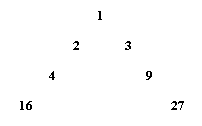A "hexagon" of numbers was chosen within Table 5 (that is, numbers chosen so that they appear to form the vertices of a hexagon, such as 8-16-24-18-9-6, with 12 in the middle) in which adjacent numbers represented the ratio of length, width, and heights of architectural spaces such as walls, ceilings, doors, etc. That this system of proportions exhibits harmony is illustrated by taking a pair of integers from a given row, e.g., 6 and 12 and interspersing it with its arithmetic and harmonic means.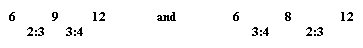Notice that the arithmetic and harmonic means insure the repetition of the ratios 2:3 and 3:4 to which they correspond . Also 2/3 x 3/4 = ½, i.e., octave is obtained by summing the intervals of a fifth and a fourth. Similar harmonic relationships hold for Tables 6 and 7. For example, place the generalized arithmetic and harmonic means between 12 and 36 in Table 6 and the common arithmetic and harmonic means between 18 and 54 in Table 7.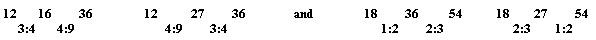We have a repetition of the ratios 3:4 and 4:9 in the first case and 1:2 and 2:3 in the second. Also, note that 3/4 x 4/9 = 1/2 x 2/3 = 1/3. This repetition of ratios holds for the general case of the 1:x sequence with the multiplication of ratios equaling 1/x.

THE ROMAN SYSTEM OF PROPORTIONS
The system of proportions based on x = q where q = 1 + Ö2 is of particular interest. An integer version of this system was used in ancient Roman architecture and was written about by Theon of Smyrna, a second century A.D. mathematician and Platonist philosopher and a contemporary of Nicomachus, in a book entitled The Mathematics Useful for Understanding Plato . This proportional system used for the design of the design of the Garden Houses of Ostia, the port city of the Roman Empire, according to a study by Donald and Carol Watts [11,13]. The key to understanding the Roman system of proportions is a geometrical construction referred to by Tons Brunés, a Danish engineer and student of ancient geometry, as the Sacred Cut. When the point of a compass is placed at one vertex of a square and an arc is swept out, the edge of the square is cut by a factor of 1/Ö2, the sacred cut. Four sacred cuts mark the vertices of a regular octagon as shown in Figure 5 and lead to a subdivision of the square into three species of rectangles: a square (S) of proportion 1:1; a square root rectangle (SR) of proportion 1:Ö2; and a rectangle of proportion 1: q, referred to as a Roman rectangle (RR).

What is noteworthy about this system is that the table of generalized means and the one of common means result in a single table rising and falling from the initiating double geometric sequence as shown in Table 8.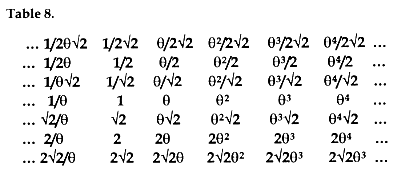Beginning with the 1: q geometric series on the central row of this table, each element below the center is the generalized arithmetic mean of the pair above it ; each element above the center is the ordinary arithmetic mean of the pair below it, e.g.,

(q + q2)/q = qÖ2 while (q + q2)/2 = q2/Ö2.

Also, each element below the center is the generalized harmonic mean of the pair below it; each element above the center is the ordinary harmonic mean of the pair above it, e.g.,

q(q)(2q)/(2+2q ) = qÖ2 while 2(q/Ö2 )(q2/Ö2 )/( q/Ö2+q2/Ö2) = q.

The algebra to carry out these operations can be seen more clearly by comparing the q-sequence with the discrete version of this sequence known as Pell's sequence.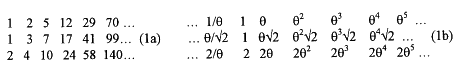Both of these triples of sequences have the Pell's sequence property : an+2 = 2an+1 + an and the ratio of successive terms of Sequences 1a approaches q in a limit sense as n® ¥. Any algebraic operation that holds for the integer sequence also holds for the q-sequence. This sequence has many additive properties, although we list only the four fundamental properties from which the others can be derived.

i) Each Pell sequence has the defining property,

 x x x Property 1: a + 2b = c , e.g., 1 + 2x2 = 5 and 1 + 2q = q2 a b c

ii,iii ) Other additive properties are,

 a b x x Property 2: a + b = d, e.g., 2 + 5 = 7 and 1 + q = q Ö2 x x Property 3: a + c = b, e.g., 2 + 3 = 5 and qÖ2 + q = q2 c d

iv) Property 4: Any element is the double of the element two rows above it.

Also, the ratio of any term from Sequence 1b to the one above it equals Ö2 while the ratio of any integer from Sequence 1a to the one above it approximates Ö2 with the approximation asymptotically approaching Ö2 as n® ¥. It should be noted that since the sum of two integers in any row equals an integer from the row above it, an infinite number of rows are needed to insure that this proportional system has additive properties.

The algebraic properties of the Roman system can be made palpable by considering the equivalent geometric properties based on the interrelation of the proportions: 1, Ö2, and q. These relationships are shown in Figure 6. For example, if S is either removed or added to SR, this results in RR, as Figure 6a illustrates. This is equivalent to Properties 2 and 3. That 2S +RR = RR is equivalent to Property 1 (see Figure 6b). Finally, if SR is cut in half it forms two SR at a smaller scale (Figure 6c), while two SR added together form an enlarged SR (see Figure 6d) as predicted by Property 4, the doubling property of Table 8.

The architectural system based on these proportions has many additive properties in which a small number of modules are able to fit together in countless ways. Figure 7 shows the breakdown of a square of dimensions q3xq3 into nine subrectangles with lengths and widths from Table 8 satisfying,

q3 = 1 + 2q + 2q + qÖ2 (2)
q3 = qÖ2 + 2q + q + qÖ2

It is a good exercise to verify Equations 2 using Properties 1-4 of the Roman system. Because of the additive properties, these rectangles can be rearranged in many ways to tile the same rectangle. This versatility is illustrated in the design in Figure 8 by Mark Bak which contains S, SR, and RR at three different scales.

All the tones of the Pythagorean scale are based on the ratio of string lengths in which the numerator and denominator are divisible by primes 2 and 3, e.g., the musical fifth, fourth, and octave: 2/3, ¾, and ½. The SR rectangle is particularly suited for creating the musical proportions of the Pythagorean scale, since it has a natural bisecting and trisecting property. By the construction of Figure 1 an oblique line drawn to the midpoint of the side of a rectangle cuts the diagonal of at the trisection point and the bisection of the SR rectangle divides it into two SR rectangles according to Figure 6c. Kim Williams has shown that the Medici Chapel was created with numerous SR rectangles enabling its design to be embedded with many musical ratios [11,14].

THE MODULOR OF LE CORBUSIER
As we mentioned, if x = f the Nicomachus sequence collapses to a single line, that of the Fibonacci sequence known as the f-sequence,

 1 f f2 f3 f4 f5 ...

where fn + fn+1 = fn+2. In order to make the f-sequence into a system of architectural proportions, the great 20th-century architect Le Corbusier cleverly adjoined a second series multiplied by 2 to form so-called "Red and Blue sequence", which he named the Modulor [15,16, 17].

Table 9.

 Blue 2/f 2 2f 2f2 2f3 2f4 2f5... Red 1 f f2 f3 f4 f5 f6 ...

Each sequence is a geometric series with ratio 1: f. Each element of one sequence fills the gap between two elements of the other sequence. Just as for Table 5 based on the ratio 1:2, each element of the Red sequence is the arithmetic mean of the pair of elements of the Blue series that brace it, while each element of the Blue series is the harmonic mean of the pair of elements from the Red series that brace it. Since the sum of a pair of integers from either the Red or Blue sequence results in another integer in that same sequence, only these two sequences are necessary to assure that the sequences have additive properties unlike the Roman system which requires an infinity of sequences.

This system also has many additive properties, which enables a rectangle to be tiled in countless ways by a small repertoire of modules. Figure 9 shows a square tiled in three ways with the same species of rectangles whose lengths and widths are numbers from the Red and Blue sequence. Figure 10 shows the exterior of Le Corbusier's Unité d'Habitation, built 1947-52 in the outskirts of Marseille, with its windows exhibiting the Modulor tiling. Additional details are presented elsewhere .

CONCLUSION
I have presented the mathematics behind the Nicomachus sequence and shown it and its generalizations to be the basis of three successful systems of proportion, the musical system of proportions of Alberti, the Roman system of proportions, and the Modulor of LeCorbusier. I have also shown that Farey series and the geometry of the Brunes star is suggested by the generalizations of Nicomachus sequences. Since Farey series is also fundamental to an understanding of a modern study of dynamical systems, we see that the voice of Nicomachus still echoes through the halls of mathematics.

APPENDIX
Proof of Nicomachus's Theorem

Let ca = (a+b)/x where b/a = x. It follows, using algebra, that,

ca = a(1+1/x) = b(1/x +1/x2). (A1)

But,

1/x + 1/x2 = 1 where f = (1+Ö5)/2. (A2)

Therefore it follows from Equations A1 and A2 that,

a < ca £ b for x ³ f

Let ch = xab/(a+b) where b/a = x. It follows, using algebra, that,

ch = a[1/(1/x+1/x2)] = b[1/(1+1/x)]. (A3)

It follows from Equations A2 and A3 that,

a £ ch < b for x ³ f

BIBLIOGRAPHY
1. Nicomachus. Introduction to Arithmetic. M.L. D'Ooge, translator. Ann Arbor: University of Michigan Press (1938).

2. Nicomachus. The Manual of Harmonics. F.R. Levin, trans. Grand Rapids, MI: Phanes Press (1994). To order this book from Amazon.com, click here.

3. Anatole Beck, Michael Bleicher and Donald Crowe. Excursions in Mathematics. The Millennium Edition. A.K. Peters, Ltd. To order this new edition of the 1969 publication, click here.

4. Jay Kappraff. Mathematics Beyond Measure: A Random Walk through Nature, Myth, and Number. Singapore:World Scientific Publ. Co., 2001 (in preparation).

5. R.A. Rasch. "Farey systems of musical intonation." Contemporary Music Review. Vol.2, pp. 31-67. (1988)

6. Jay Kappraff. "The structure of Ancient Musical Scales with a Modern Twist." In preparation.

7. Tons Brunés. The Secrets of Ancient Geometry and its Use. Copenhagen: Rhodos Press (1967).

8. Jay Kappraff. "A Secret of Ancient Geometry." Geometry at Work. MAA Notes 53. Cathy Gorini, et al., eds. Mathematical Association of America Press (2000).

9. J. Kapusta. Private communication

10. Rudolph Wittkower. Architecture in the Age of Humanism. New York: Norton (1971). To order the newest (1998) edition of this classic book, click here.

11. Jay Kappraff. "Musical Proportions at the Basis of Systems of Architectural Proportion both Ancient and Modern." Nexus: Architecture and Mathematics. Kim Williams, ed. Fucecchio, Italy: Edizioni Dell'Erba (1996). To order this book from Amazon.com, click here.

12. Theon of Smyrna. The Mathematics Useful for Understanding Plato. R. and D. Lawlor, trans. Sand Diego: Wizard's Bookshelf (1979). To order this book from Amazon.com, click here.

13. Donald J. and Carol Martin Watts. "The Garden Houses of Ostia." Scientific American (Dec. 1986).

14. Kim Williams. "Michelangelo's Medici Chapel: The Cube, the Square, and the Root-2 Rectangle." Leonardo. vol. 30, no. 2 (1997), pp. 105-112.

15. Jay Kappraff. Connections: The Geometric Bridge between Art and Science. New York: McGraw-Hill (1991).

16. Kappraff, Jay. "Linking the Musical Proportions of Renaissance, the Modulor, and Roman Systems of Proportions." Space Structures, Vol. 11, Nos. 1 and 2 (1996).

17. Le Corbusier. Modulor and Modulor 2. Basel: Birkhauser (2000). To order this book from Amazon.com, click here.

18. Joseph Rykwert, Neil Leach, Robert Tavernor. Leon Battista Alberti. On the Art of Building in Ten Books. Cambridge: MIT Press (1988). To order this book from Amazon.com, click here.

RELATED SITES ON THE WWW

Jay Kappraff holds a Ph.D. from the Courant Institute of Mathematical Science at New York University. He is an associate professor of mathematics at the New Jersey Institute of Technology where he has developed a course in the mathematics of design for architects and computer scientists. Prior to that, he taught at the Cooper Union College in New York City and held the position of aerospace engineer at NASA. He has published numerous articles on such diverse subjects as fractals, phyllotaxy, design science, plasma physics, passive solar heating and aerospace engineering. He has also lectured widely on the relationships between art and science. He is the author of Connections: The Geometric Bridge Between Art and Science. His new book, Mathematics Beyond Measure: A Random Walk Through Nature, Myth and Number, is to be published soon.

 The correct citation for this article is:Jay Kappraff, "The Arithmetic of Nicomachus of Gerasaand its Applications to Systems of Proportion", Nexus Network Journal, vol. 2, no. 4 (October 2000), http://www.nexusjournal.com/Kappraff.html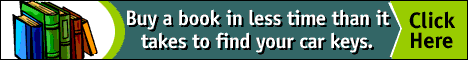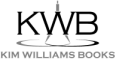top of page

NNJ Homepage

Related Sites on the WWW

Order books!

Research Articles

The Geometer's Angle

Didactics

Book Reviews

Conference and Exhibit Reports